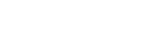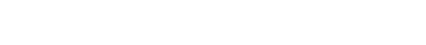`# 判断各变量中是否存在缺失值data3.isnull().any(axis = 0)# 各变量中缺失值的数量data3.isnull().sum(axis = 0)# 各变量中缺失值的比例data3.isnull().sum(axis = 0)/data3.shape``# 判断数据行中是否存在缺失值`

`# 缺失观测的行数data3.isnull().any(axis = 1).sum()# 缺失观测的比例data3.isnull().any(axis = 1).sum()/data3.shape``# 删除字段 -- 如删除缺失率非常高的edu变量data3.drop(labels = 'edu', axis = 1, inplace=True)# 数据预览data3.head()``# 删除观测，-- 如删除age变量中所对应的缺失观测data3_new = data3.drop(labels = data3.index[data3['age'].isnull()], axis = 0)# 查看数据的规模data3_new.shapeout:(2900, 5)`

`# 替换法处理缺失值data3.fillna(value = {'gender': data3['gender'].mode(), # 使用性别的众数替换缺失性别                 'age':data3['age'].mean() # 使用年龄的平均值替换缺失年龄                 },          inplace = True # 原地修改数据          )# 再次查看各变量的缺失比例data3.isnull().sum(axis = 0)``# 读取数据titanic = pd.read_csv('Titanic.csv')# 删除缺失严重的Cabin变量titanic.drop(labels='Cabin', axis = 1, inplace=True)# 根据Embarked变量，删除对应的缺失行titanic.dropna(subset=['Embarked'], inplace=True)# 删除无关紧要的变量（这些变量对后面预测年龄没有太多的帮助）titanic.drop(labels=['PassengerId','Name','Ticket','Embarked'], axis = 1, inplace=True)# 将字符型的性别变量映射为数值变量titanic.Sex = titanic.Sex.map({'male':1, 'female':0})# 将数据拆分为两组，一是年龄缺失组，二是年龄非缺失组，后续基于非缺失值构建KNN模型，再对缺失组做预测nomissing = titanic.loc[~titanic.Age.isnull(),]missing = titanic.loc[titanic.Age.isnull(),]# 导入机器学习的第三方包from sklearn import neighbors# 提取出所有的自变量X = nomissing.columns[nomissing.columns != 'Age']# 构建模型knn = neighbors.KNeighborsRegressor()# 模型拟合knn.fit(nomissing[X], nomissing.Age)# 年龄预测pred_age = knn.predict(missing[X])`